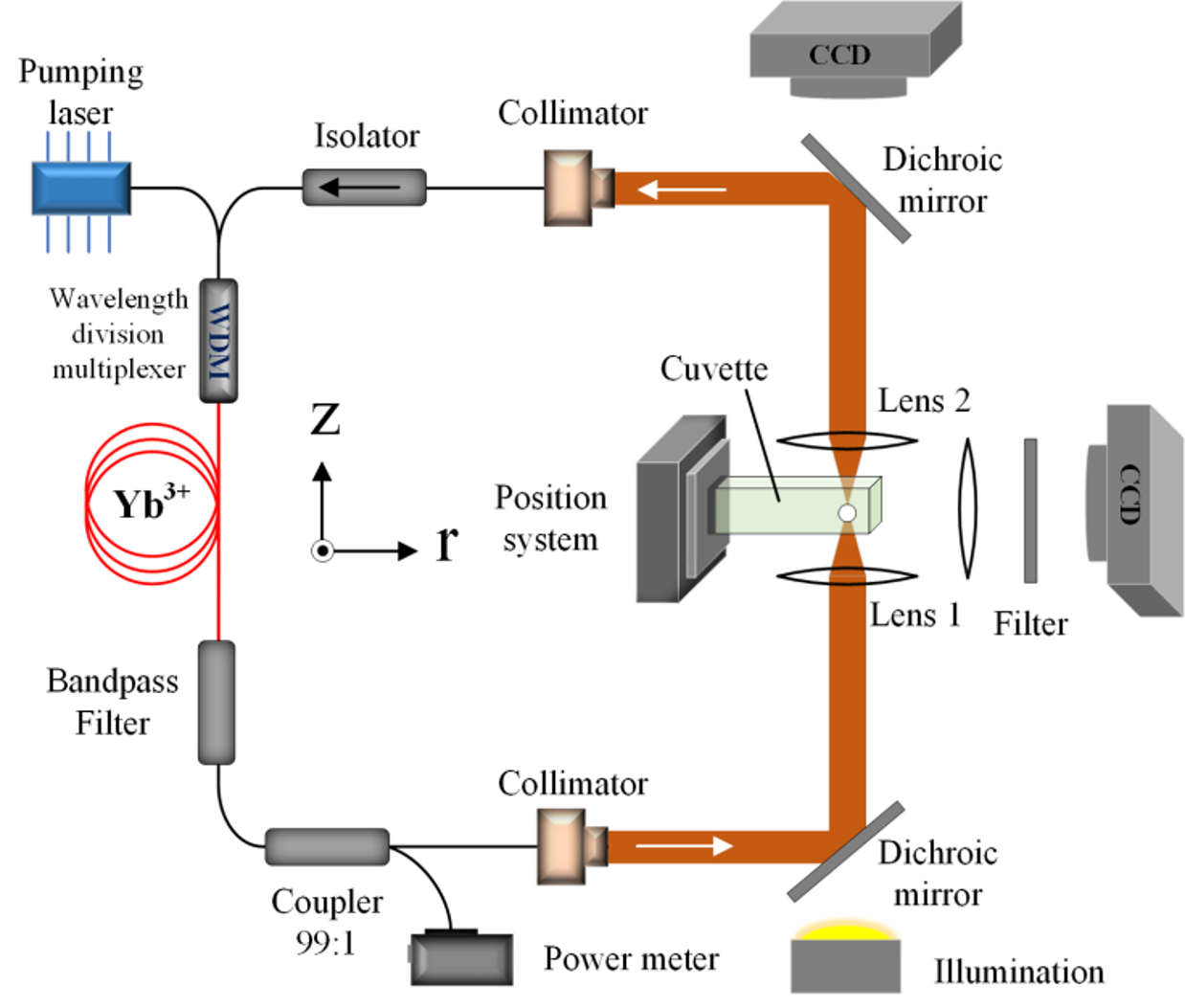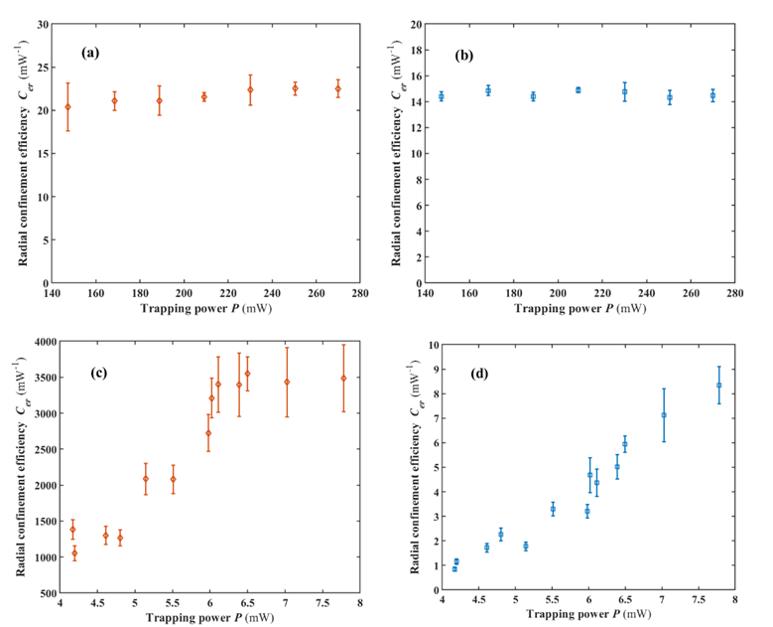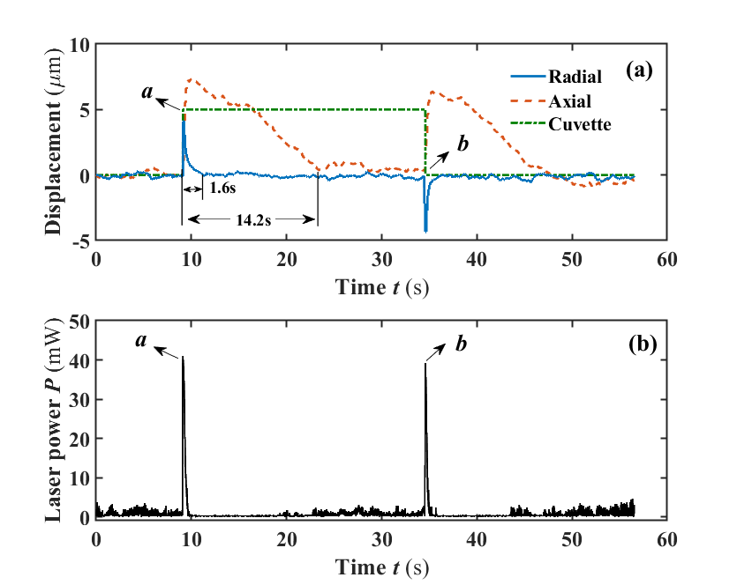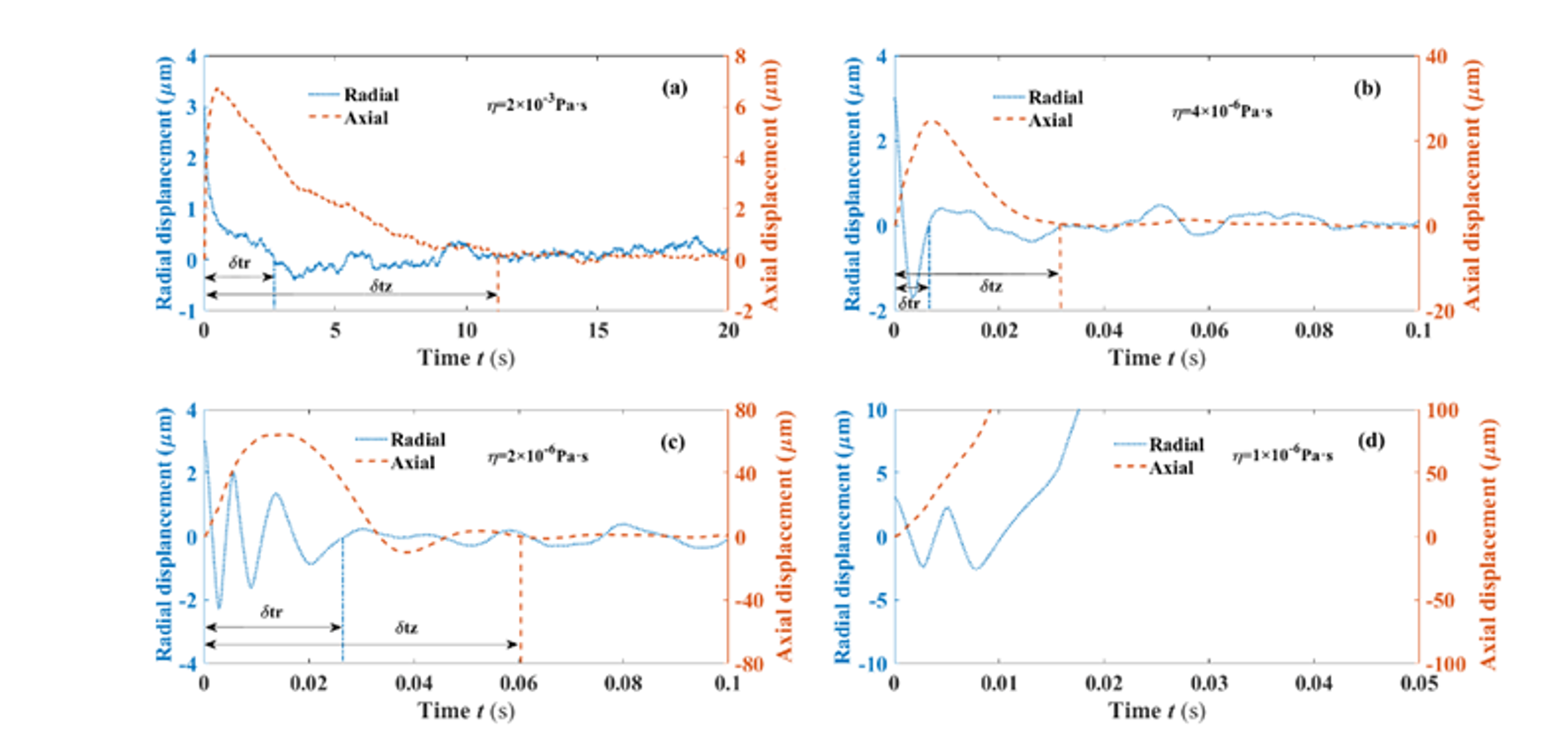+86
+86

×

+86

×$S_{r}=\left [\frac{1}{n}\sum_{n}\left (r_{i}-\overline{r} \right )^{2} \right ]^{-1},S_{z}=\left [\frac{1}{n}\sum_{n}\left (z_{i}-\overline{z} \right )^{2} \right ]^{-1}$ $C_{er}=S_{r}/I_{t}$https://www.osapublishing.org/oe/abstract.cfm?uri=oe-27-25-36653